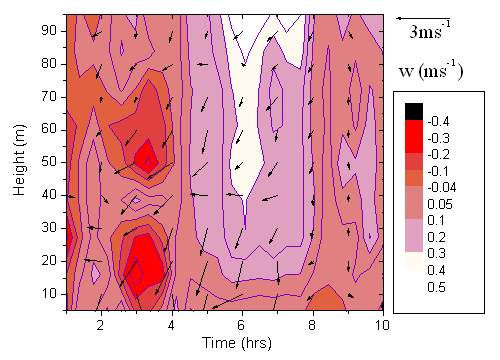Skip Navigation Links.

### Wind Analysis Results Plotted as a Contour Plot with Vector Plot OverlayDescription of the Graph

Three-dimensional velocity data in the lower part of the atmosphere is presented. The data obtained for this graph was recorded with an acoustic sounder for a day. A vector plot is used to present wind speed and wind direction data. The magnitude (length) of each vector in the graph represents wind speed while the angle (rotation) of each vector represents wind direction. The long arrow in the top right corner of the graph represents a wind from the east with wind speed 3 ms-1. The Contour Color Fill plot is used to represent vertical velocities as a function of time and height. Positive values indicate upward motion and negative values indicate downward motion. This graph let's us understand the variation of three dimensional wind with height.

Description of How the Plot was Created Using Origin

The raw vertical velocity data was processed externally and then imported into a matrix in Origin. The data was then plotted as a 'Contour Color Fill' plot. The legend on the right side of the plot represents the vertical velocity scale. Wind speed and direction were also externally processed and then imported into a worksheet in Origin (as X, Y, Angle, and Magnitude data). This data was then plotted as a 'Vector XYAM' plot. Both plots were then merged and superimposed. The axes of both plots were indentically scaled to match and the axes from one layer were then hidden.

Biography

Thara V. Prabha is a post doctoral associate with University of Georgia, GA, USA. She is working on theoretical simulations of canopy atmosphere exchange. The main emphasis of her current work is to study turbulence characteristics inside and above forest canopy. The study is quite relevant to ongoing efforts to monitor the gas exchange between the atmosphere and vegetation. Thara has been using Origin since 1994.

Thara V. Prabha
Lab for Environmental Physics, University of Georgia
Redding building, 1109 Experiment Street, Griffin, GA 30223